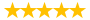# bio145 lab 5

Question # 00004206 Posted By: mac123 Updated on: 11/27/2013 06:13 PM Due on: 11/29/2013
Subject Biology Topic General Biology Tutorials:
Question

1. a. What are the three classes of mollusks? (3 points)

b. Explain the shells of each of the three classes of mollusks. (3 points)

c. Name one example of each of the three classes of mollusks. (3 points)

2. What is the largest class of mollusks? (1 point)

3. Name one cephalopod that still has a shell. (1 point)

4. Why is the name cephalopod appropriate for this class of animals? (1 point)

5. a. What does it mean to exhibit bilateral symmetry? (1 point)

b. Which side of a squid is the dorsal side? (1 point)

c. Which side of a squid is the ventral side? (1 point)

6. a. How many tentacles are found on a squid? (1 point)

b. What is the function of the tentacles? (1 point)

c. How many arms are there on a squid? (1 point)

d. What is the function of the arms? (1 point)

e. How is the arrangement of suckers on the tentacles different from the arrangement on the arms? (1 point)

7. a. How does a squid maintain body stability? (1 point)

b. How does a squid propel itself through the water? (1 point)

8. How does a male squid transfer sperm from its body to that of the female? (1 point)

9. a. How do squid bring oxygen into their bodies? (1 point)

b. How do they get rid of carbon dioxide? (1 point)

10. Identify the functions of the following structures: (10 points)

a. beak

b. eye

c. ganglia

d. hearts

e. mantle

f. pen

h. stomach

i. suckers

j. testes

11. What is the olfactory crest? (1 point)

12. What are two ways that squid can protect themselves from predators? (2 points)

13. The pen is the vestigial internal shell of the squid. What does the term vestigial mean? (1 point)

14. a. What is an advantage of having a shell? (1 point)

b. What is an advantage of not having a shell? (1 point)

15. How many species of cephalopods are found in freshwater? (1 point)

17. How many hearts does a squid have? (1 point)

19. What are chromatophores? (1 point)

20. a. Was the squid male or female? (1 point)

b. What was the distinguishing characteristic? (1 point)

Tutorials for this Question
1. ## Solution: bio145 lab 5

Tutorial # 00003998 Posted By: mac123 Posted on: 11/27/2013 06:15 PM
Puchased By: 3
Tutorial Preview
The solution of bio145 lab 5...
Attachments
bio145_lab5.docx (20.59 KB)
Recent Feedback
Rated By Feedback Comments Rated On
b...osReasonable prices and secure payment 05/11/2016

Great! We have found the solution of this question!